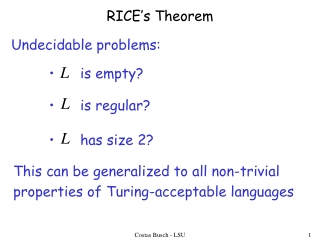DownloadDownload PresentationRICE’s Theorem

# RICE’s Theorem

Télécharger la présentation## RICE’s Theorem

- - - - - - - - - - - - - - - - - - - - - - - - - - - E N D - - - - - - - - - - - - - - - - - - - - - - - - - - -
##### Presentation Transcript

1. RICE’s Theorem Undecidable problems: • is empty? • is regular? • has size 2? This can be generalized to all non-trivial properties of Turing-acceptable languages Costas Busch - LSU

2. Non-trivial property: A property possessed by some Turing-acceptable languages but not all : is empty? Example: YES NO NO Costas Busch - LSU

3. More examples of non-trivial properties: : is regular? YES YES NO : has size 2? NO NO YES Costas Busch - LSU

4. Trivial property: A property possessed by ALL Turing-acceptable languages Examples: : has size at least 0? True for all languages : is accepted by some Turing machine? True for all Turing-acceptable languages Costas Busch - LSU

5. We can describe a property as the set of languages that possess the property If language has property then : is empty? Example: YES NO NO Costas Busch - LSU

6. Example: Suppose alphabet is : has size 1? NO YES NO NO Costas Busch - LSU

7. Non-trivial property problem Input: Turing Machine Question: Does have the non-trivial property ? Corresponding language: Costas Busch - LSU

8. Rice’s Theorem: is undecidable (the non-trivial property problem is unsolvable) Proof: Reduce (membership problem) to or Costas Busch - LSU

9. We examine two cases: Case 1: Examples: : is empty? : is regular? Case 2: Example: : has size 2? Costas Busch - LSU

10. Case 1: Since is non-trivial, there is a Turing-acceptable language such that: Let be the Turing machine that accepts Costas Busch - LSU

11. Reduce (membership problem) to Costas Busch - LSU

12. membership problem decider Decider for Non-trivial property problem decider Reduction YES YES Compute Decider NO NO Given the reduction, if is decidable, then is decidable A contradiction! since is undecidable Costas Busch - LSU

13. We only need to build the reduction: Reduction Compute So that: Costas Busch - LSU

14. Construct from : Tape of input string Turing Machine Accept yes yes • Write on tape, and accepts ? • Simulate on input Costas Busch - LSU

15. For this we can run machine , that accepts language , with input string Turing Machine Accept yes yes • Write on tape, and accepts ? • Simulate on input Costas Busch - LSU

16. accepts does not accept Turing Machine Accept yes yes • Write on tape, and accepts ? • Simulate on input Costas Busch - LSU Prof. Busch - LSU 16

17. Therefore: accepts Equivalently: Costas Busch - LSU

18. Case 2: Since is non-trivial, there is a Turing-acceptable language such that: Let be the Turing machine that accepts Costas Busch - LSU

19. Reduce (membership problem) to Costas Busch - LSU

20. membership problem decider Decider for Non-trivial property problem decider Reduction YES YES Compute Decider NO NO Given the reduction, if is decidable, then is decidable A contradiction! since is undecidable Costas Busch - LSU

21. We only need to build the reduction: Reduction Compute So that: Costas Busch - LSU

22. Construct from : Tape of input string Turing Machine Accept yes yes • Write on tape, and accepts ? • Simulate on input Costas Busch - LSU

23. accepts does not accept Turing Machine Accept yes yes • Write on tape, and accepts ? • Simulate on input Costas Busch - LSU

24. Therefore: accepts Equivalently: END OF PROOF Costas Busch - LSU